• os.path.join(path1[, path2[, ...]])：把目录和文件名合成个路径 os.path.exists(path)：路径是否存在 os.mkdir(path[, mode])：创建目录 import os file_path = './log/Server.txt' parent_path = os.path.abs....
os.path.abspath(path)：绝对路径 os.path.join(path1[, path2[, ...]])：把目录和文件名合成一个路径 os.path.exists(path)：路径是否存在 os.mkdir(path[, mode])：创建目录
import os

file_path = './log/Server.txt'
parent_path = os.path.abspath(os.path.join(file_path, ".."))
if os.path.exists(parent_path) == False:
os.makedirs(parent_path)

with open(file_path, 'w', encoding='utf-8') as f:
f.write("Hello World!")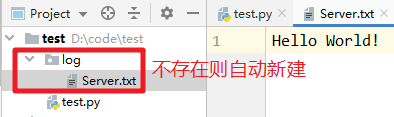参考文献
Python os.path() 模块
展开全文• 下面小编就为大家分享一篇python3获取当前文件上一级目录实例，具有很好的参考价值，希望对大家有所帮助。一起跟随小编过来看看吧
• python3 如何 获取一个文件目录,获取 上一级目录 1 _file_ 是什么 ? if __name__ == '__main__': file = __file__ print(f"file = {__file__!r}") 结果如下 file = ‘C:/Users/changfx/PycharmProjects/...
python3 如何 获取一个文件的目录,获取 上一级目录
1 _file_ 是什么 ?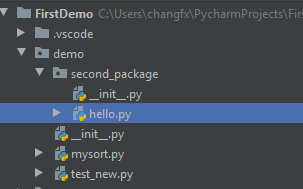# hello.py

if __name__ == '__main__':
file = __file__
print(f"file = {__file__!r}")


结果如下
file = ‘C:/Users/changfx/PycharmProjects/FirstDemo/demo/second_package/hello.py’
结果 是打印 file 的 路径
python datamodel.html
# -*- coding: utf-8 -*-
"""
#    看下 __file__  这个变量的含义 ，

https://docs.python.org/3/reference/datamodel.html

__file__ is the pathname of the file from which the module was loaded, if it was loaded from a file.
The __file__ attribute may be missing for certain types of modules,
such as C modules that are statically linked into the interpreter;
for extension modules loaded dynamically from a shared library, it is the pathname of the shared library file.

"""

说明：

在 python 中 __file__ 是一个文件路径， 当 一个python 模块 被加载的时候， 它是从file load 进来的。 它是一个属性。 它是一个特殊的属性，这个属性就代表了 这个文件的路径。 按照官方文档的方法，这个 __file__ 属性 有可能 没有对于 某些特定的模块类型， 比如说 C 模块，它是直接 链接到解释器 。 对于从共享库动态加载的扩展模块，它是共享库文件的路径名。

    from os.path import dirname
path = "C:/Users/changfx/PycharmProjects/FirstDemo/demo/second_package/hello.py"

print(dirname(path))
print(dirname(dirname(path)))

print(dirname(dirname(dirname(path))))
print(dirname(dirname(dirname(dirname(path)))))


从这里 就可以 获取 不同的目录 ，dirname 就可以拿到当前 文件所在的目录 ， 多次使用 就可以 继续 拿到 上一级 目录 。
结果如下：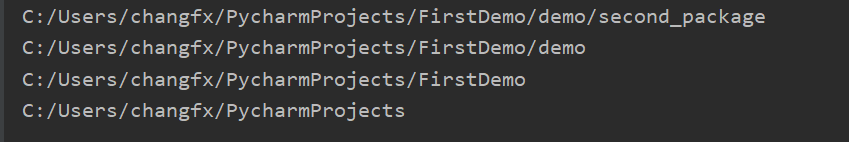import os
from os.path import dirname

def get_path():
print('=== file ===')
print(__file__)

print('\n===获取当前目录 ===')
print(os.path.abspath(dirname(__file__)))

print("\n ===获取 当前文件的 上一级目录 === ")
# get package dir
package_dir = os.path.abspath(dirname(dirname(__file__)))
print(package_dir)

print("\n ===获取 当前文件的 上上一级目录 === ")
# print(os.path.abspath(os.path.join(os.getcwd(), "../..")))
print(os.path.abspath(dirname(dirname(dirname(__file__)))))


结果如下图：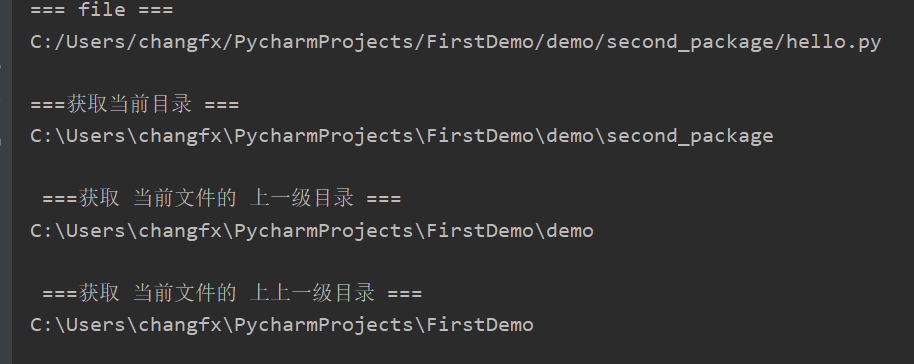2 如何获取文件的目录
如何获取文件的目录 以及 上一级目录，上上一级目录
可以封装几个函数 来获取 这些文件路径 。
还是以这个项目结构 为例， 写一个 hello.py# -*- coding: utf-8 -*-
# hello.py

from os.path import dirname
from os.path import abspath

def get_current_dir():
"""
获取 当前文件所在 目录
:return:
"""

return abspath(dirname(__file__))
pass

def get_parent_dir():
"""
获取当前 文件的 目录 的 上一级 目录
:return:
"""
pass
return abspath(dirname(dirname(__file__)))

def get_double_parent_dir():
"""

获取当前 文件的目录 的 上一级 目录 的 父目录

:return:
"""
pass
return abspath(dirname(dirname(dirname(__file__))))

if __name__ == '__main__':
print(get_current_dir())
print(get_parent_dir())
print(get_double_parent_dir())

结果如下：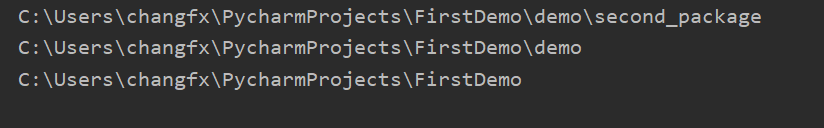参考文档
stackoverflow https://stackoverflow.com/questions/9271464/what-does-the-file-variable-mean-do

分享快乐,留住感动. 2019-09-30 18:57:19 --frank


展开全文• 当前py文件为当前目录下的python.py 二 获取当前目录 上级目录 上级目录 import os print('***获取当前目录***') print("当前目录是:{}".format(os.getcwd())) print("当前目录是:{}".format(os.path....
一 目录层级关系如下图
当前py文件为当前目录下的python.py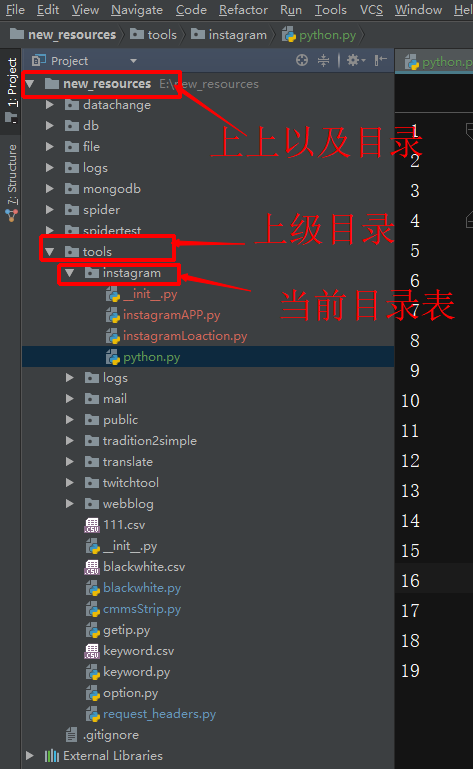二 获取当前目录  上级目录  上上级目录
import os

print('***获取当前目录***')
print("当前目录是:{}".format(os.getcwd()))
print("当前目录是:{}".format(os.path.abspath(os.path.dirname(__file__))))

print('***获取上级目录***')
print("上级目录是:{}".format(os.path.abspath(os.path.dirname(os.path.dirname(__file__)))))
print("上级目录是:{}".format(os.path.abspath(os.path.dirname(os.getcwd()))))
print("上级目录是:{}".format(os.path.abspath(os.path.join(os.getcwd(), ".."))))

print('***获取上上级目录***')
print("上上级目录是:{}".format(os.path.abspath(os.path.join(os.getcwd(), "../.."))))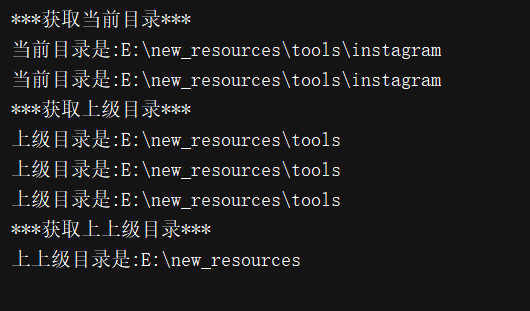展开全文• 获取文件上一级目录 parent_dir = os.path.dirname(os.path.dirname(os.path.realpath(__file__)))
from os.path import realpath, dirname

# 获取当前文件的全路径名
file_path = os.path.realpath(__file__)

# 上一级目录
parent_dir = os.path.dirname(file_path)

# 上上级目录
parent_dir = os.path.dirname(os.path.dirname(file_path))

展开全文• 文件所在目录上一级目录 os.path.abspath(os.path.join(os.path.dirname('settings.py'),os.path.pardir))   os.path.pardir是父目录 os.path.abspath是绝对路径
• 因为使用python+selenium有时候需要获取当前文件上一级目录，找了一段时间找到了，在此记录下来； os.path.dirname(os.path.dirname(os.path.abspath(__file__)))这个是获取当前文件上一级目录 sys.path....
• 文件所在目录上一级目录 os.path.abspath(os.path.join(os.path.dirname('settings.py'),os.path.pardir)) os.path.pardir是父目录 os.path.abspath是绝对路径
• python获取文件上一级目录：取文件所在目录上一级目录 os.path.abspath(os.path.join(os.path.dirname(‘settings.py’),os.path.pardir)) os.path.pardir是父目录，os.path.abspath是绝对路径 举例具体看一下输出...os
• 需求：给个大的路径，想要筛选包含特定文件的文件夹目录，文件夹下子文件夹内的东西不做检索。 import os from natsort import natsorted g = os.walk(r'g:\test') # 获取所有文件及子文件 List = [] for path,...
• import os # 获取当前目录 print(os.getcwd()) print(os.path.abspath(os.path.dirname(__file__))) ...# 获取当前文件 print(os.path.abspath(__file__)) # 获取上级目录 print(os.path.abspath(os.path....
• print('***获取当前目录***') print(os.getcwd()) print(os.path.abspath(os.path.dirname(__file__))) print('***获取上级目录***') print(os.path.abspath(os.path.dirname(os.path.dirname(__file__)))) print(o...路径
• **原文：**...python获取文件上一级目录：取文件所在目录上一级目录 os.path.abspath(os.path.join(os.path.dirname(‘settings.py’),os.path.pardir)) os.path.pardir是父目录，os.path....文件夹操作
• print '***获取当前目录***' print os.getcwd() print os.path.abspath(os.path.dirname(__file__)) print '***获取上级目录***' print os.path.abspath(os.path.dirname(os.path.dirname(__file__))) print os....
• # 当前文件不是文件夹，获取文件后缀 file_type = os.path.splitext(each_path) # 设置个flag，标志当前文件类型和要移动的文件类型是否一致 selected = False # 如果一致或者没有给出指定类型，标志量为true ...
• python中，import module会去sys.path搜索，sys.path是个列表，并且我们可以动态修改。...既然这样，要import上一级目录的module,可以sys.path.insert(0,parentdir)。 不过这种写绝对路径的方式，如import path
• 获取文件路径实现 1.1 获取当前文件路径 import os current_file_path = __file__ print(f"current_file_path: {current_file_path}") __file__变量其实有个问题，当文件被是被调用文件时__file__总是文件的...
• 假如当前文件绝对路径：E:\learn\python\我的file\my.py#coding:utf-8 import os #dirName：上级目录名称 #sysCoding：系统编码格式 #targetCoding：转换目标编码格式 def get_dir_realpath(dirName,sysCoding,...绝对路径 算法
• import上一级目录的模块 python中，import module会去sys.path搜索，sys.path是个列表，并且我们可以动态修改。...所以用动态方法来获取上一级目录。 import os,sys parentdir = os.path.dirname(os.path.di
• #coding=UTF-8 import os ...# 上一级路径（父级路径） parent_path = os.path.dirname(current_path) print("当前路径：" + current_path) print("上一级路径：" + parent_path) ...path
• 一、获取当前文件路径二、获取上一级文件路径这里可以想象出获取与自己同级文件的路径，这么写：即先获取上级目录，然后通过join函数来把同级的目录test拼进来。三、获取当前路径下的所有文件名得到的是一个列表的...python当前路径
• 学习并总结使用Python获取当前文件路径以及上一级路径的方法（os模块）。 学习os模块基本知识，本次会使用：os.getcwd()、os.path.dirname、os.path.abspath()、os.pardir、os.path.pardir、os.path、join()； 准备...os
• #coding=utf-8 import os #当前 print os.getcwd() print os.path.abspath(os.path.dirname(__...#上一级 print os.path.abspath(os.path.dirname(os.path.dirname(__file__))) print os.path.abspath(os.path.joi...
• Python的os模块提供函数可以获取到当前文件的绝对路径，从而定位到目标文件。 func_path = os.path.dirname(__file__) # 获取当前脚本所在目录的绝对路径 base_dir = os.path.dirname(func_path...
• python获取当前文件所在路径的方式有以下几种： 第种是获取当前文件的全路径： # 获取当前文件的路径，包括文件名 print(__file__) 第二种是获取当前文件所在目录： # dirname去掉文件名，返回目录 ......

# python获取文件上一级目录python 订阅